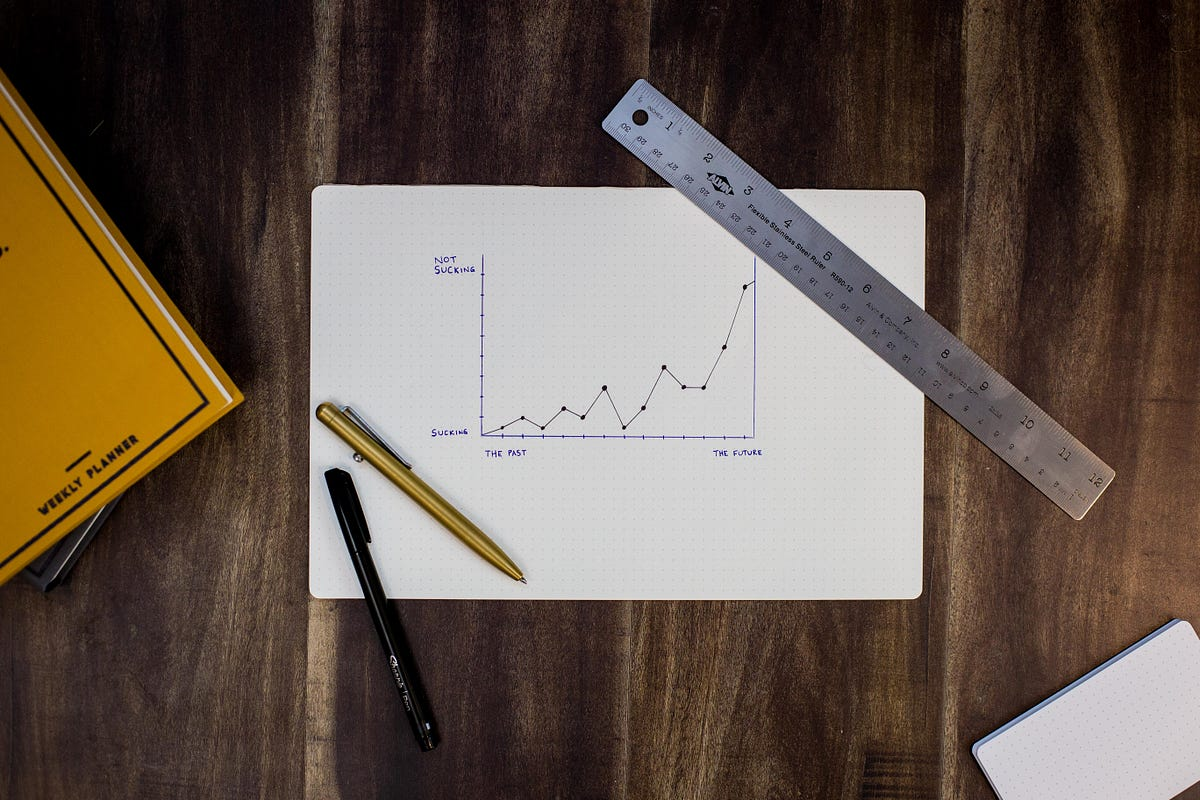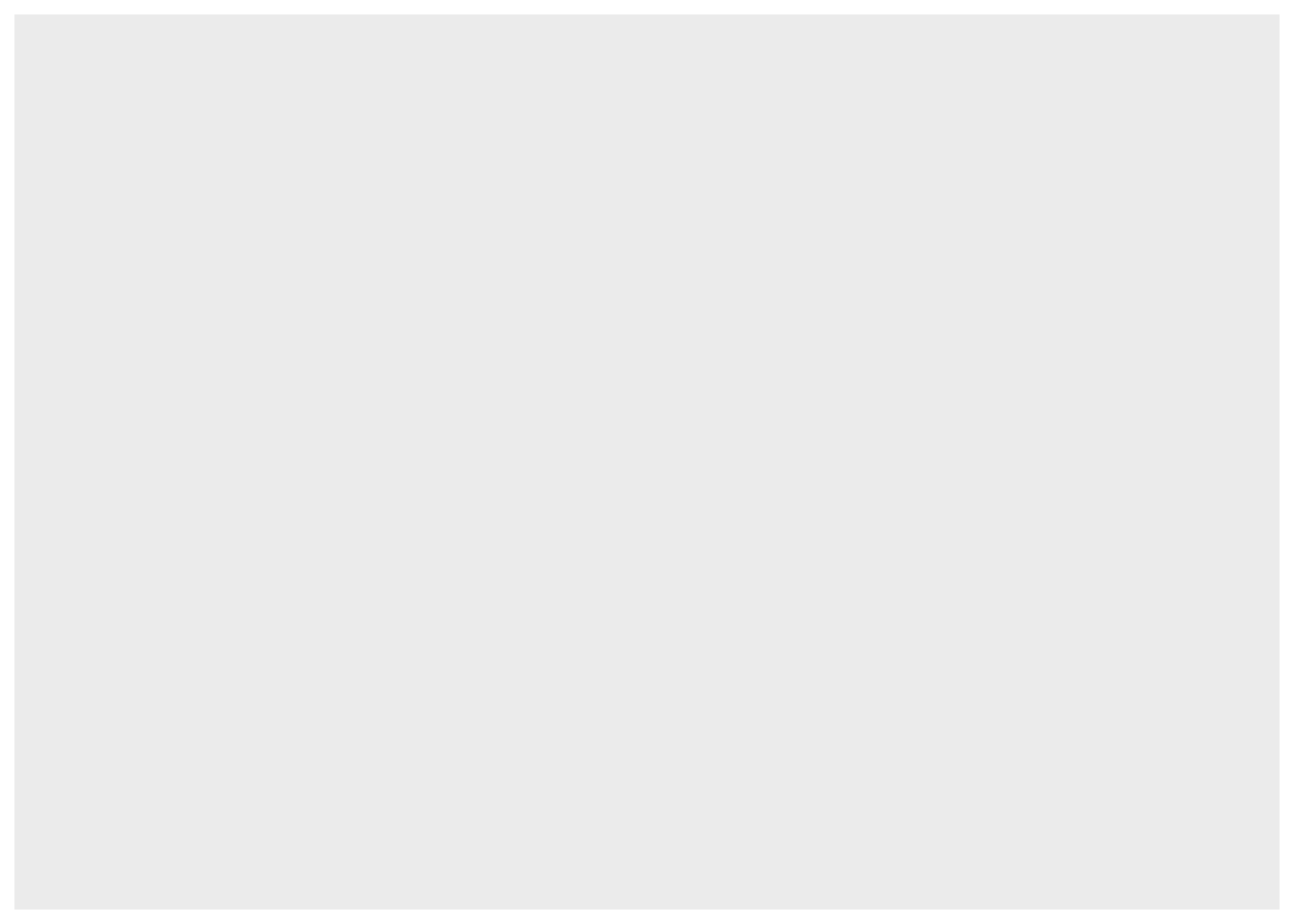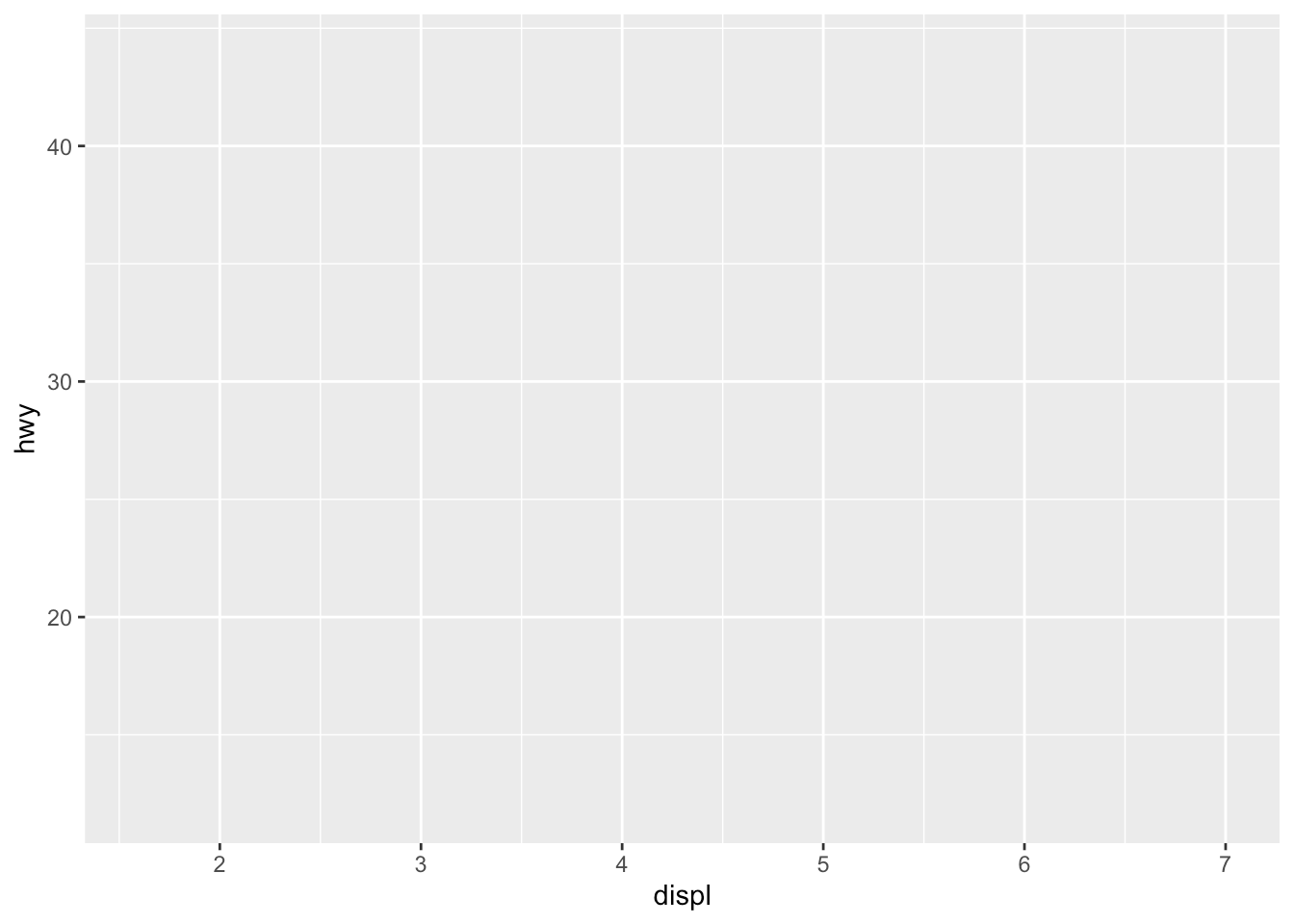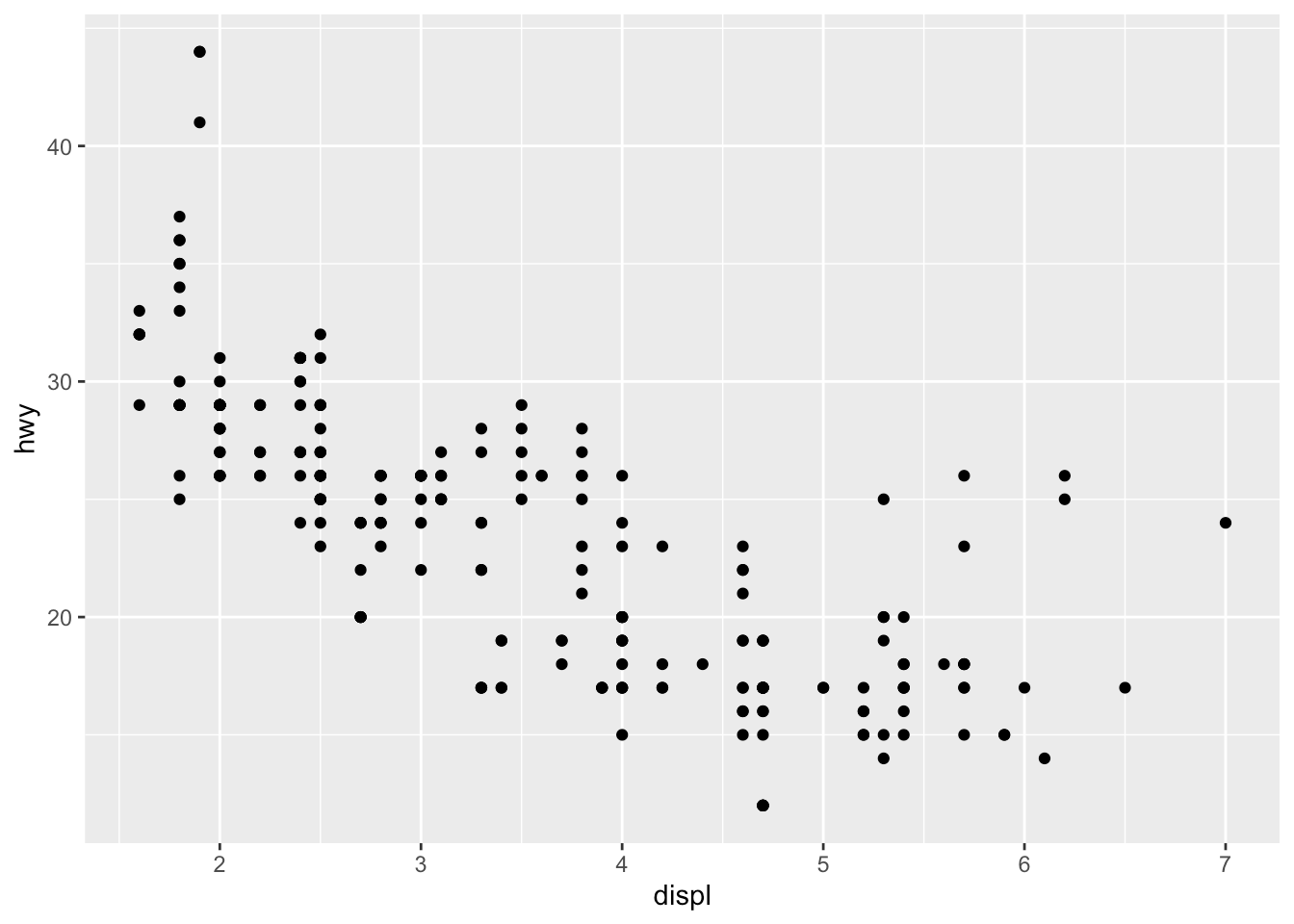# Graphics in R with ggplot2Ris known to be a really powerful programming language when it comes to graphics and visualizations (in addition to statistics and data science of course!).

## Introduction

Ris known to be a really powerful programming language when it comes to graphics and visualizations (in addition to statistics and data science of course!).

To keep it short, graphics in R can be done in two ways, via the:

1. `{graphics}` package (the base graphics in R, loaded by default)
2. `{lattice}` package which adds more functionalities to the base package
3. `{ggplot2}` package (which needs to be installed and loaded beforehand)

The `{graphics}` package comes with a large choice of plots (such as `plot``hist``barplot``boxplot``pie``mosaicplot`, etc.) and additional related features (e.g., `abline``lines``legend``mtext``rect`, etc.). It is often the preferred way to draw plots for most R users, and in particular for beginners to intermediate users.

Since its creation in 2005 by Hadley Wickham, `**{ggplot2}**` has grown in use to become one of the most popular R packages and the most popular package for graphics and data visualizations. The `{ggplot2}` package is a much more modern approach to creating professional-quality graphics. More information about the package can be found at ggplot2.tidyverse.org.

In this article, we will see how to create common plots such as scatter plots, line plots, histograms, boxplots, barplots, density plots in R with this package. If you are unfamiliar with any of these types of graph, you will find more information about each one (when to use it, its purpose, what does it show, etc.) in my article about descriptive statistics in R.

## Data

To illustrate plots with the `{ggplot2}` package we will use the `mpg` dataset available in the package. The dataset contains observations collected by the US Environmental Protection Agency on fuel economy from 1999 to 2008 for 38 popular models of cars (run `?mpg` for more information about the data):

``````library(ggplot2)
dat <- ggplot2::mpg``````

Before going further, let’s transform the `cyl``drv``fl``year` and `class` variables in factor with the `transform()` function:

``````dat <- transform(dat,
cyl = factor(cyl),
drv = factor(drv),
fl = factor(fl),
year = factor(year),
class = factor(class)
)``````

## Basic principles of `{ggplot2}`

The `{ggplot2}` package is based on the principles of “The Grammar of Graphics” (hence “gg” in the name of `{ggplot2}`), that is, a coherent system for describing and building graphs. The main idea is to design a graphic as a succession of layers.

The main layers are:

1. The dataset that contains the variables that we want to represent. This is done with the `ggplot()` function and comes first.
2. The variable(s) to represent on the x and/or y-axis, and the aesthetic elements (such as color, size, fill, shape and transparency) of the objects to be represented. This is done with the `aes()` function (abbreviation of aesthetic).
3. The type of graphical representation (scatter plot, line plot, barplot, histogram, boxplot, etc.). This is done with the functions `geom_point()``geom_line()``geom_bar()``geom_histogram()``geom_boxplot()`, etc.
4. If needed, additional layers (such as labels, annotations, scales, axis ticks, legends, themes, facets, etc.) can be added to personalize the plot.

To create a plot, we thus first need to specify the data in the `ggplot()` function and then add the required layers such as the variables, the aesthetic elements and the type of plot:

``````ggplot(data) +
aes(x = var_x, y = var_y) +
geom_x()``````
• `data` in `ggplot()` is the name of the data frame which contains the variables `var_x` and `var_y`.
• The `+` symbol is used to indicate the different layers that will be added to the plot. Make sure to write the `+` symbol at the end of the line of code and not at the beginning of the line, otherwise R throws an error.
• The layer `aes()` indicates what variables will be used in the plot and more generally, the aesthetic elements of the plot.
• Finally, `x` in `geom_x()` represents the type of plot.
• Other layers are usually not required unless we want to personalize the plot further.

Note that it is a good practice to write one line of code per layer to improve code readability.

## Create plots with `{ggplot2}`

In the following sections we will show how to draw the following plots:

• scatter plot
• line plot
• histogram
• density plot
• boxplot
• barplot

In order to focus on the construction of the different plots and the use of `{ggplot2}`, we will restrict ourselves to drawing basic (yet beautiful) plots without unnecessary layers. For the sake of completeness, we will briefly discuss and illustrate different layers to further personalize a plot at the end of the article (see this section).

Note that if you still struggle to create plots with `{ggplot2}` after reading this tutorial, you may find the {esquisse} addin useful. This addin allows you to interactively (that is, by dragging and dropping variables) create plots with the `{ggplot2}` package. Give it a try!

## Scatter plot

We start by creating a scatter plot using `geom_point`. Remember that a scatter plot is used to visualize the relation between two quantitative variables.

1. We start by specifying the data:
``ggplot(dat) ## data``2. Then we add the variables to be represented with the `aes()` function:

``````ggplot(dat) + ## data
aes(x = displ, y = hwy) ## variables``````3. Finally, we indicate the type of plot:

``````ggplot(dat) + ## data
aes(x = displ, y = hwy) + ## variables
geom_point() ## type of plot``````You will also sometimes see the aesthetic elements (`aes()` with the variables) inside the `ggplot()` function in addition to the dataset:

``````ggplot(mpg, aes(x = displ, y = hwy)) +
geom_point()``````This second method gives the exact same plot than the first method. I tend to prefer the first method over the second for better readability, but this is more a matter of taste so the choice is up to you.

## Data Cleaning in R for Data Science

A data scientist/analyst in the making needs to format and clean data before being able to perform any kind of exploratory data analysis.

## R For Data Science Full Course | Data Science With R Full Course |Data Science Tutorial

Learn the essential concepts in data science and understand the important packages in R for data science. You will look at some of the widely used data science algorithms such as Linear regression, logistic regression, decision trees, random forest, including time-series analysis. Finally, you will get an idea about the Salary structure, Skills, Jobs, and resume of a data scientist.

## Data Science With R Training in Hyderabad | Data Science Courses in Hyderabad

Best Data Science With R Training in Hyderabad - We Provides Best Data Science Certification Courses in Hyderabad offering extensive Data Science With R Training by Data scientists. Enrol Today!

## 50 Data Science Jobs That Opened Just Last Week

Data Science and Analytics market evolves to adapt to the constantly changing economic and business environments. Our latest survey report suggests that as the overall Data Science and Analytics market evolves to adapt to the constantly changing economic and business environments, data scientists and AI practitioners should be aware of the skills and tools that the broader community is working on. A good grip in these skills will further help data science enthusiasts to get the best jobs that various industries in their data science functions are offering.

## Statistics for Data Science

Statistics for Data Science and Machine Learning Engineer. I’ll try to teach you just enough to be dangerous, and pique your interest just enough that you’ll go off and learn more.